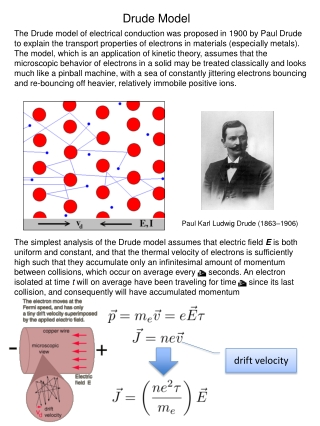Download PresentationDrude Model

# Drude Model - PowerPoint PPT PresentationDownload Presentation## Drude Model

- - - - - - - - - - - - - - - - - - - - - - - - - - - E N D - - - - - - - - - - - - - - - - - - - - - - - - - - -
##### Presentation Transcript

1. Drude Model The Drude model of electrical conduction was proposed in 1900 by Paul Drude to explain the transport properties of electrons in materials (especially metals). The model, which is an application of kinetic theory, assumes that the microscopic behavior of electrons in a solid may be treated classically and looks much like a pinball machine, with a sea of constantly jittering electrons bouncing and re-bouncing off heavier, relatively immobile positive ions. The simplest analysis of the Drude model assumes that electric field E is both uniform and constant, and that the thermal velocity of electrons is sufficiently high such that they accumulate only an infinitesimal amount of momentum between collisions, which occur on average every t seconds. An electron isolated at time t will on average have been traveling for time t since its last collision, and consequently will have accumulated momentum Paul Karl Ludwig Drude (1863–1906) drift velocity

2. Ohm’s Law conductivity resistivity: Georg Simon Ohm (1789–1854) If the potential difference between the ends is V, what current flows? resistance: (measured in Ohms=V/A)

3. The work done by the electrical force is converted into heat in the resistor. Joule heating law Series and parallel circuits Series circuit resistors in series Parallel circuit resistors in parallel

4. RC circuit B A A) B)